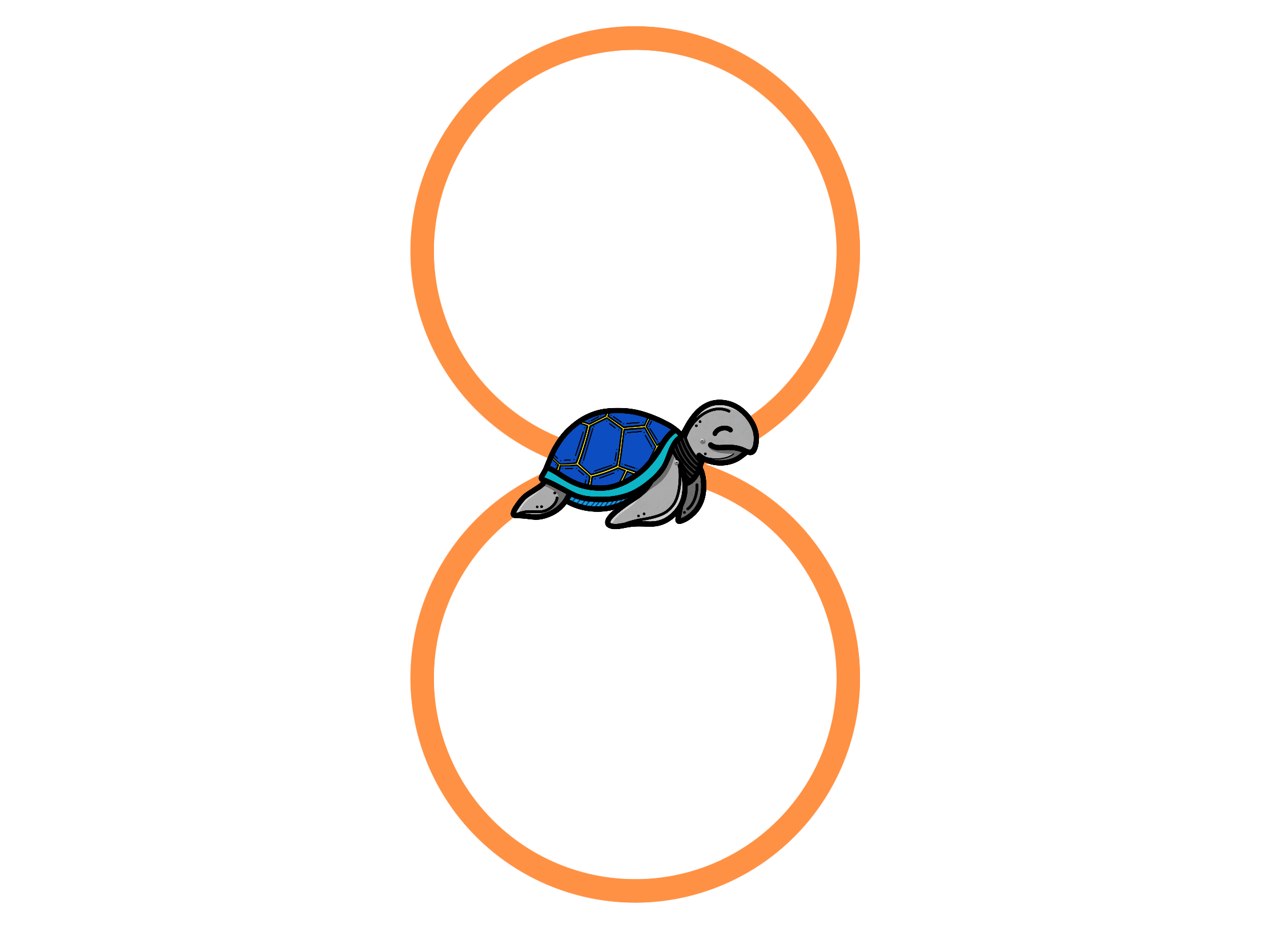# Activity 7 - Drawing a figure 8Great! Now let’s make a figure 8 as the first step to making a mandala flower! A figure 8 is just two circles that are opposite to each other. To do this we can make use of the turtle.circle(x) method, which takes an integer x as the radius of the circle. (You may recall that 2 * radius is how wide the circle is.) Having the radius as an argument means we can decide how big or small the circle should be.

  import turtle
turtle.circle(20)


In the above example, the circle is 40 pixels wide and the circle is drawn in a counterclockwise direction. If the argument to turtle.circle() is negative, then the circle is drawn in a clockwise direction and it is opposite to the original version.

Using this information, create a new function called figure_8() which draws a figure 8.